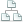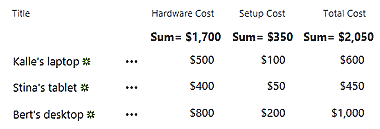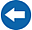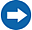Home >Tips >SharePoint Workflows >Totals of rows and columnsSite map

# Vertical and Horizontal Totals Calculation

## A SharePoint Workflows tutorial by Peter KalmströmThe SharePoint,Totals? feature can be used to summarize values in a SharePoint column, and the result of the calculation is shown on top of the column that has been calculated. This is a very useful feature but it has two problems:
• The Totals view cannot be used in modern lists.
• The Totals view cannot be used on calculated columns.
Only Microsoft can solve the UI issue, but a a workflow can solve the problem with the calculated column.

In the demo below Peter Kalmström, CEO and Systems Designer of kalmstrom.com Business Solutions, shows how to build a workflow that calculates the sum of values in two currency columns and adds it to a third currency column. As we are not using a calculated column, we can add the Totals view to all three columns and get both vertical and horizontal sums.### Calculate sums workflow

A list item?s,Total Cost??? column cannot be updated with a calculation directly in an,Update Item? action. Instead, Peter uses a local variable that gets its value from a,Do Calculation? action.
1. Create a new SharePoint 2013 workflow that is run every time an item is created or changed. Go to the end of the workflow.
2. Add the action 'Do Calculation' for the sum of the two values that should be summarized. Output the result to a new variable.
3. Add the action 'Set Field in Current Item' and set the field to the total cost. Set the value to the variable you created in the previous step.
4. Check, publish and test the workflow.